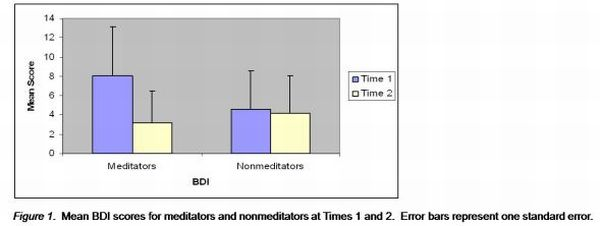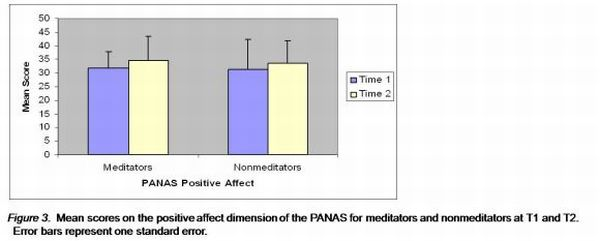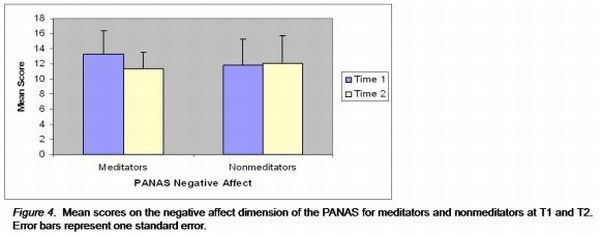# Change on Self-Report Measures of Affect

### Richard Chambers

To determine whether the meditation course successfully enhanced participants' affect in the predicted directions (Hypothesis 1), participants' scores before and after the intervention (Time 1 [T1] and Time 2 [T2], respectively) on the BDI, BAI and PANAS (positive and negative affect dimensions) were analysed by two-way 2x2 ANOVA procedures with factors of time (T1 vs. T2) and group (meditators vs. nonmeditators). All indices were examined for missing values and to ensure they satisfied the assumptions of ANOVA (Gravetter & Wallnau, 2000). No missing values were found, and the assumptions of homogeneity of variance, normality, and independence of observations were upheld.

BDI. Figure 1 represents the mean BDI scores, for each group, at T1 and T2. The meditators demonstrated a highly significant decrease over the course of the intervention (t = 6.66, p < .001, d = 1.13), while the nonmeditators demonstrated no significant decrease (t = .63, p = .54, d = .28) .ANOVA analysis revealed that there was a highly significant main effect of time (F[1,38] = 27.14, p < .001, â•¬Ã€2 = 4.17), a nonsignificant main effect of group (F[1,38] = 1.04, p = .32, â•¬Ã€2 = .03), and a highly significant time by group interaction effect (F[1,38] = 18.78, p < .001, â•¬Ã€2 = .33).

BAI. Figure 2 represents the mean BAI scores, for each group, at T1 and T2. The meditators demonstrated a highly significant decrease over the course of the intervention (t = 2.81, p = .01, d = .70), while the nonmeditators demonstrated a trend toward a significant decrease (t = 1.74, p = .10, d = .34).There was a highly significant main effect of time (F[1,38] = 10.96, p = .002, â•¬Ã€2 = .22), a nonsignificant main effect of group (F[1,38] = 2.00, p = .17, â•¬Ã€2 = .05), and a nonsignificant time by group interaction effect (F[1,38] = 2.31, p = .14, â•¬Ã€2 = .06).

PANAS Positive Affect. Figure 3 represents the mean PANAS Positive Affect scores, for each group, at T1 and T2. The meditators demonstrated no significant increase over the course of the intervention (t = -1.45, p = .16, d = .07), as did the nonmeditators (t = -1.55, p = .14, d = -.71).There was a significant main effect of time (F[1,38] = 4.47, p = .04, â•¬Ã€2 = .11), a nonsignificant main effect for group (F[1,38] = .09, p = .76, â•¬Ã€2 = .03) and a nonsignificant time by group interaction (F[1,38] = .004, p = .95, â•¬Ã€2 < .001).

PANAS Negative Affect. Figure 4 represents the mean PANAS Negative Affect scores, for each group, at T1 and T2. The meditators demonstrated a highly significant decrease over the course of the intervention (t = 3.88, p = .001, d = .69), while the nonmeditators demonstrated no significant decrease (t = -.38, p = .71, d = -.06).There was a near-significant main effect of time (F[1,38] = 3.89, p = .06, â•¬Ã€2 = .09), a nonsignificant main effect of group (F[1,38] = .19, p = .67, â•¬Ã€2 = .005), and a significant time by group interaction effect (F[1,38] = 6.69, p = .01, â•¬Ã€2 = .15).